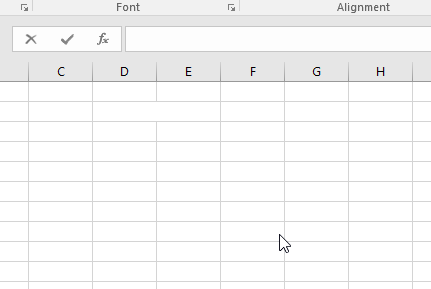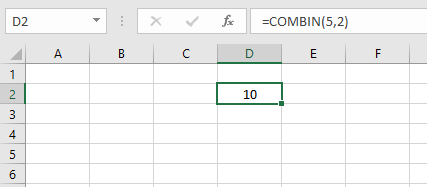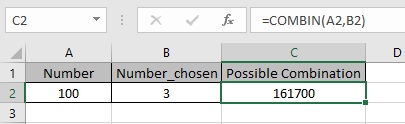# How to use the COMBIN Function in Excel

Combination is a mathematical operator for getting the number of possible combinations having number (n) & number chosen (r).
Cnr= FACT(n) / [FACT(n-r) * FACT(r)]
OR
Cnr= (n)! / (n-r)!*(r)!
Factorial function in excel is called FACT function and its mathematical symbol is ! .

The COMBIN function returns the value which is the number of possible combinations. Order is not significant in combinations.
Syntax:

=COMBIN (number, number_chosen)

Number : total number of elements or values
Number_chosen : number of values chosen from the total values.
All of this might be confusing so let’s Gear up and understand the COMBIN function using it in an example.

If there are 10 numbers stated 1 to 10. And you get the total number ways selecting any one number from 1 to 10 can be calculated using the COMBIN function.There are 10 ways i.e. 10 possible ways to select one number from the combination of 10.
Possible values :
1 2 3 4 5 6 7 8 9 10
Now Let’s consider vowels . There are 5 vowels in the alphabet which are a, i, e, o & u
And we need to select 2 vowels out of 5 vowels.
So we need to use the formula to get the combinations of two vowels out of five

=COMBIN(5, 2)

5 : five vowels
2 : two vowels combination requiredThere are 10 possible ways which are
ae ai ao au = 4
ei ei eu = 3
io iu = 2
ou = 1
Total = 10
Order of vowels is not significant when using the COMBIN function. If order is significant use the Excel PERMUT function.

Now take a few examples to get the possible combination having bigger numbers.There are 161700 possible combinations when three different numbers chosen out of 100.

Note:

• Arguments that contain decimal values are truncated to integers.
• The COMBIN function returns a #VALUE! error value if either argument is not numeric.
• If number is less than number_chosen, the function returns #NUM! error.

As you can get from the above examples that the COMBIN function returns the total number of possible combinations of choosing numbers out of total numbers.

Hope you understood how to use COMBIN function in Excel. Explore more articles on Excel PERMUT function here. Please feel free to state your query or feedback for the above article.

Related Article:

How to use the PERMUT Function in Excel

How to use the FACT Function in Excel

Popular Articles:

50 Excel Shortcuts to Increase Your Productivity

How to use the VLOOKUP Function in Excel

How to use the COUNTIF in Excel 2016

How to use the SUMIF Function in Excel

Terms and Conditions of use

The applications/code on this site are distributed as is and without warranties or liability. In no event shall the owner of the copyrights, or the authors of the applications/code be liable for any loss of profit, any problems or any damage resulting from the use or evaluation of the applications/code.